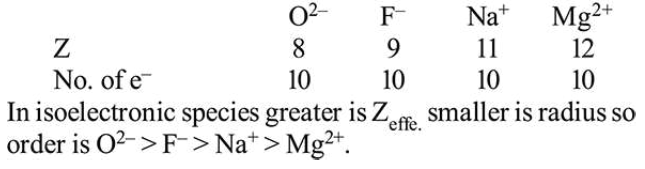Question:

The ionic radii of $\mathrm{O}^{2-}, \mathrm{F}^{-}, \mathrm{Na}^{+}$and $\mathrm{Mg}^{2+}$ are in the order:

1. $\mathrm{F}^{-}>\mathrm{O}^{2-}>\mathrm{Na}^{+}>\mathrm{Mg}^{2+}$

2. $\mathrm{O}^{2-}>\mathrm{F}^{-}>\mathrm{Na}^{+}>\mathrm{Mg}^{2+}$

3. $\mathrm{Mg}^{2+}>\mathrm{Na}^{+}>\mathrm{F}^{-}>\mathrm{O}^{2-}$

4. $\mathrm{O}^{2-}>\mathrm{F}^{-}>\mathrm{Mg}^{2+}>\mathrm{Na}^{+}$

Correct Option: , 2

Solution: